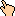## 1995-12 Solution

1. Two. It's exactly 29/16 = 1 13/16, which is pretty close to two.
2. 5050. Note that 1 + 100 = 2 + 99 = 3 + 98, etc, = 101. That's 50 of them, since the last one is 50 + 51, so 101 * 50 = 5050. Those who had infinite series' in school probably recognized the sum as n (n + 1) / 2, which when you plug 100 in for n, gives exactly 101 * 50 = 5050.
3. 6. The pattern 2,4,8,6,2,etc. continues on forever so all that's needed is where 312 fits into the pattern. The pattern repeats with a frequency of 4. 312 mod 4 = 0, which is the 6 in the pattern (same as 4, 8, 12, etc.). I.e. 1sDigit(24) = 1sDigit(28) = ... = 1sDigit(2312) = 6.

WWW Maven: Dan Garcia (ddgarcia@cs.berkeley.edu)Send me feedback